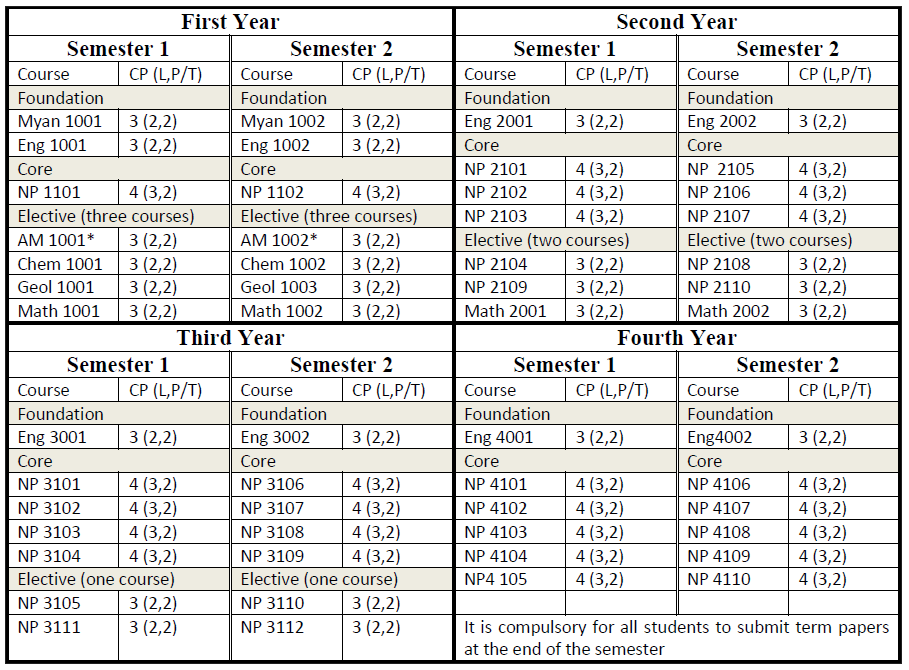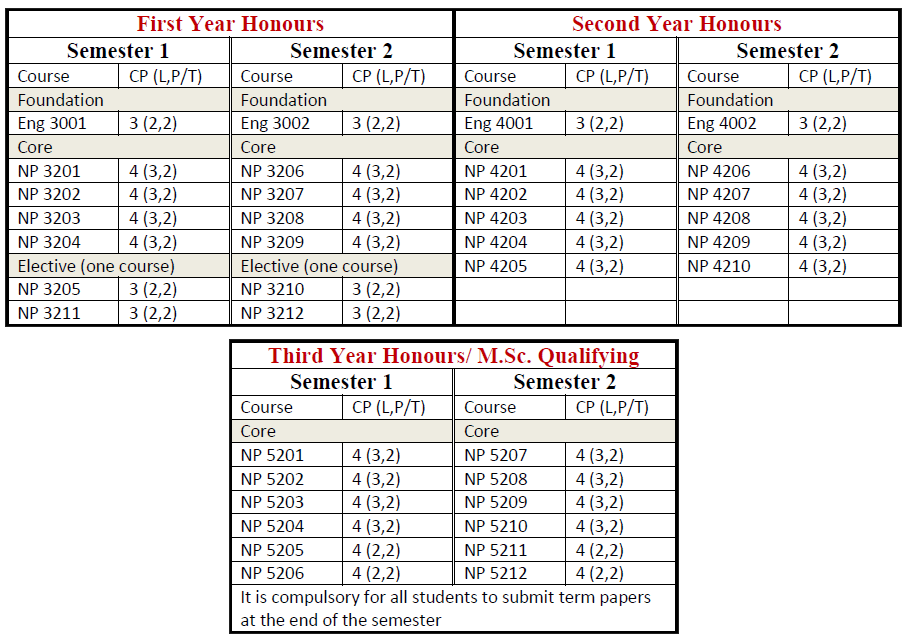# Dagon University

## ဒဂုံတက္ကသိုလ်

### Curriculum

#### B.Sc. in Nuclear Physics#### B.Sc. (Honours) in Nuclear Physics

Students who passed second year with GPA 4 or GPA greater than 4 are eligible to attend B.Sc. (Honours) classes for three more years. After finished successfully, they earn B.Sc. (Hons) degree specializing Nuclear Physics.### Course Descriptions

#### NP 1101: General Physics

The module covers Motion in two and three dimensions, Force and Motion I, Force and Motion II, Work and Kinetic Energy, Conservation of energy, Systems of particles, collisions, rotation, rolling, torque and angular momentum, equilibrium and elasticity, oscillations, Waves I, and Waves II.

#### NP 1102: Modern Physics

The module deals with Special Relativity, Wave-Particle Duality, Rutherford model of atom, -particle scattering, Rutherford scattering formula, The Bohr Model of the Atom, Quantum Mechanics and Atoms, The Nucleus and Radioactivity, Fission, Fusion and Elementary particles.

#### NP 2101: Atomic and Nuclear Physics I

This module is concerned with Nuclear Dimensions, Electron orbits, Atomic spectra, The Bohr atom, Energy levels and spectra, Correspondence principle, and Nuclear Motion.

#### NP 2102: Electromagnetic Theory I

The module includes Coulomb’s law, The electric Field; Gauss’s Law, Potential, Capacitance, Properties of Dielectrics, Current, resistance and electromotive force, Direct- Current Circuits and Instruments, The magnetic Field, Magnetic Forces on Current-Carrying Conductors, Magnetic Field of a current, Induced Electromotive force, Inductance, and Magnetic Properties of Matter.

#### NP 2103: Physical Optics

This module focuses on Image formed by a Single Surface, Lenses and Optical Instruments, Interference and Diffraction, Polarization.

#### NP 2104: Vector and Tensor Analysis

The module deals with Vector Algebra, Matrices and Vector Space, Vector Calculus, Line Surface and Volume Integrals, Tensors, Calculus of Variations.

#### NP 2105: Atomic and Nuclear Physics II

The module covers Nuclear Structure, Radioactive decay, Radioactivity,  and  decay, Radioactive series, Energy and nuclear reaction, Atomic bomb, Fission reactor, Fusion reactor, and Nucleosynthesis.

#### NP 2106: Electromagnetic Theory II

This module familiarises students with Maxwell’s Equations, Propagation of Electromagnetic Wave, The Nature and Propagation of light, and Waves in Bounded Regions.

#### NP 2107: Laser Physics

The module covers The Amplification of light, Optically Pumped Laser, Optical Resonators, and Specific Laser Systems.

#### NP 2108: Differential Equation

The module provides students with Series and limit, Ordinary and Partial Differential Equations, Physical Applications.

#### NP 2109: Statistical Mechanics

The module includes Microstates and degeneracy, distinguishable and indistinguishable particles, Thermodynamic functions of state, distribution functions, Entropy, disorder and the probability of system configuration occurring, and Information theory. It also covers Arrow of time, Equilibrium configuration, Boltzmann distribution, Temperature, Partition function, One-dimensional harmonic oscillator, Planck distribution, Black body radiation, Fermi-Dirac and Bose- Einstein distributions.

#### NP 2110: Relativistic Mechanics

This module deals with Lorentz Transformations, Phase velocity, wave velocity, group velocity, Relativistic kinetic energy, Length, Mass and Time in Special Relativity, and Addition of velocities. It also includes Energy moment-four vectors, The twin paradox, Introduction to curved space-time, and Gravitation as curved space-time phenomenon.

#### NP 3101/ 3102: Theoretical Nuclear Physics IA

The module covers Internucleon Forces I, Nucleon forces, Scattering experiments, Bound state, Experimental results, Proton-proton forces, Nuclear Moments and Nuclear Shape. It also includes Electromagnetic multipoles, Electric moments, Magnetic moments, Electromagnetic moments of the deutron and tensor forces.

#### NP 3102/ 3202: Applied Nuclear Physics IA

This module is concerned with Interaction of charged particles with matter, Law of absorption and emission, photoelectric effect, Compton scattering, pair production, and Energy loss of charged particles due to ionization.

#### NP 3103/ 3203: Mathematical Physics I

The module enables students to be familiar with ODE, PDE, Series solutions of ordinary differential equations, Special Functions, and The Dirac delta function.

#### NP 3104/ 3204: Condensed Matter Physics I

The module enables students to understand Crystal Structure, Reciprocal Lattice, Crystal Binding and Elastic Constants, Phonons I, and Crystal Vibrations.

#### NP 3105/ 3205: AC Circuit

The module deals with Pure R circuit, Pure L circuit, Pure C circuit, RL series circuit, RC series circuit, RLC series circuit.

#### NP 3106/ 3206: Theoretical Nuclear Physics IB

This module covers Nuclear Binding energies, The semi-empirical mass formula, Magic numbers, other mass formula, and Introduction to Nuclear Models.

#### NP 3107/ 3207: Applied Nuclear Physics IB

The module deals with Gas filled counter; Scintillation counter, Cerenkov counter; Solid state detector, GM counter, Nuclear emulsions, technique of measurement and analysis of tracks; Proton synchrotron, linear accelerations, and Acceleration of heavy ions.

#### NP 3108/ 3208: Quantum Mechanics I

This module covers Historical review, The Schrödinger Wave Equation, Eigen functions and Eigen values, Discrete Eigen values.

#### NP 3109/ 3209: Condensed Matter Physics II

The module focuses on Phonons II, Thermal Properties, Free Electron Fermi Gas, Energy Bands, and Semiconductor Crystals.

#### NP 3110/ 3210: Introduction to Electronics

The module covers Electrical Quantities, Circuit Principles, Signal Waveforms, Physical Electronics, Alternating Current Circuits, Introduction to Semiconductor, Diode application, and Special Purpose Diodes. It also includes Bipolar Junction Transistors, Transistors Bias Circuits, Small-Signal Bipolar Amplifiers, Power Amplifiers, Field Effect Transistors and Biasing Small-Signal FET Amplifiers, Thyristors and Other Devices, and Operational Amplifiers.

#### NP 3111/ 3211: Classical Mechanics

The module deals with Mechanics of a system of particles and constraints, The generalized coordinates. It includes D’Alembert’s principle and Lagrange’s equation, Euler Lagrange eqn, Hamilton’s principle, Hamilton’s principle for a conservative system, Principle of least action, Hamilton’s equations, Ignorable coordinates, Characteristic Functions and Hamilton-Jacobi Eqn, Special transformations, Lagrange Brackets, and Poison’s brackets.

#### NP 3112/ 3212: Mathematical Physics II

This module familiarises students with Complex Numbers, Functions of a complex variable, Gamma and Beta functions, Bessel function, and Differentiation under the integral sign.

#### NP 4101/ 4201: Theoretical Nuclear Physics IIA

The module covers Partial wave analysis, Single – particle model, Extreme single–particle model and spin, Configuration mixing, Correlation in nuclear matter, Introduction to the Brueckner method or the individual-pair model, and Collective nuclear motion.

#### NP 4102/ 4202: Applied Nuclear Physics IIA

The module is concerned with Photon(Gamma ray and X-ray)Spectroscopy, and Charge particle Spectroscopy.

#### NP 4103/ 4203: Mathematical Physics III

The module enables students to be familiar with Complex variable, Functions of a complex variable, Cauchy-Riemann conditions, Cauchy integral theorem, Cauchy integral formula, The residue theorem, and evaluation of integrals using residue theorem.

#### NP 4104/ 4204: Statistical Physics I

The module focuses on Equilibrium and State Quantities, The Laws of Thermodynamics, Phase Transition and Chemical Reactions, and Thermodynamic Potentials.

#### NP 4105/ 4205: Nuclear Instrumentation and Nucleonic I

This module covers Introduction, The Detector, The NIM Concept, The High-Voltage Power Supply, The Preamplifier, The Amplifier, The Oscilloscope, The Discriminator or Single-Channel Analyzer (SCA), The Scaler, The Timer, and The Multichannel Analyzer (MCA).

#### NP 4106/ 4206: Theoretical Nuclear Physics IIB

The module deals with Collective modes of motion, Coupling of particle and collective motions, Weak coupling, Strong coupling, Particle states in distorted nuclei, Calculation of equilibrium shape, Levels of distorted odd-A nuclei, Values of inertial parameters, and Comparison of nuclear models.

#### NP 4107/ 4207: Applied Nuclear Physics IIB

The module covers Activation Analysis, Neutron Detection and Spectroscopy.

#### NP 4108/ 4208: Quantum Mechanics

This module enables students to understand Continuous Eigenvalues, Matrix Formulation of Quantum Mechanics, and Approximation Methods for Bound State

#### NP 4109/ 4209: Statistical Physics II

The module is concerned with The fundamental principles of Statistical Physics, Ideal Gases, Maxwell Distribution, The Fermi and Bose distributions.

#### NP 4110/ 4210: Nuclear Instrumentation and Nucleonics IB

The module covers Nucleonics, Introduction, Resistance, Capacitance, Inductance and Impedance, A Differentiating Circuit, An Integrating Circuit, Delay Lines, Pulse Shaping, and Timing. It also includes The Leading-Edge Timing Method, The Zero-Crossing Timing Method, The Constant-Fraction Timing Method, Coincidence-Anticoincidence Measurements, Pulse Shape Discriminator, Preamplifiers, amplifiers, and Multiparameter Analyzer.

#### NP 5201: Theoretical Nuclear Physics IIIA

This module deals with Nuclear Reaction: Neutron Physics and Nuclear Energy.

#### NP 5202: Applied Nuclear Physics IIIA

The module is concerned with Health Physics Fundamentals: Special Topics.

#### NP 5203: Quantum Mechanics IIIA

The module covers Fundamental Concepts, the Stern–Garlach Experiment, Kets, Bras and Operators, Bras Kets and Matrix Representations, Measurements, Observables and the Uncertainty Relations, Change of basis, Position, and Momentum and translation. It also includes Wave Functions in position and momentum space, Quantum Dynamics, Time Evolution and the Schrödinger equation, The Schroedinger Versus the Heisenberg Picture, propagators and Feynnman Path Integrals, Potential and Gauge Transformations.

#### NP 5204: Condensed Matter Physics III

The module provides students with Band Theory of Solids: The Bloch Theorem, The Kronig-Penney Model, Velocity and effective Mass of Electron, Distinction Between Metals, Insulators and Semiconductors, Semiconductors: Superconductivity.

#### NP 5205: Computer Programming

The module focuses on PSEUDO language and FORTRAN (77 or 90) language.

#### NP 5206: Nuclear Instrumentation & Nucleonic IIA

This module familiarises students with Detector Electronics and Pulse Processing, Pulse Processing and Shaping, and Linear and Logic Pulse Functions.

#### NP 5207: Theoretical Nuclear Physics IIIB

The module is concerned with Elementary Particles and Particle Physics; Cosmic rays.

#### NP 5208: Applied Nuclear Physics IIIB

The module deals with Nuclear Reactors and Nuclear Power: Neutron Diffusion and Moderation.

#### NP 5209: Quantum Mechanics IIIB

The module covers Theory of angular momentum, and Symmetry in Quantum mechanics.

#### NP 5210: Materials Science

This module includesProperties of materials: Electrical properties of materials, Thermal properties of materials, Optical properties of materials, Solar cell: The solar resources, Photovoltaic, Generating of solar power, Designing solar cell, and Nanotechnology: Introduction to Nanotechnology, The Science of Nanotechnology, The Nanotechnology Tool Box.

#### NP 5211: Numerical Analysis

The module deals with Locating Roots of equations, Interpolation and numerical differentiation, Numerical Integration, Trapezoidal Rule, Simpsons Scheme, and Gaussian Quadrature Formulae. It is also concerned with Systems of Linear Equations, Gaussian Elimination, Iterative Solution of Linear Equation, and FORTRAN (77 or 90) Language.

#### NP 5212: Nuclear Instrumentation & Nucleonics IIB

The module covers Multichannel Pulse Analysis.